• 矩阵 在数学中，矩阵（Matrix）是一个按照长方阵列排列的复数或实数集合。矩阵是高等代数学中的常见工具，也常见于统计分析等应用数学学科中...矩阵运算在科学计算中非常重要 ，而矩阵的基本运算包括矩阵的加法，减法，
矩阵
在数学中，矩阵（Matrix）是一个按照长方阵列排列的复数或实数集合。矩阵是高等代数学中的常见工具，也常见于统计分析等应用数学学科中。 在物理学中，矩阵于电路学、力学、光学和量子物理中都有应用；计算机科学中，三维动画制作也需要用到矩阵。 矩阵的运算是数值分析领域的重要问题。将矩阵分解为简单矩阵的组合可以在理论和实际应用上简化矩阵的运算。对一些应用广泛而形式特殊的矩阵，例如稀疏矩阵和准对角矩阵，有特定的快速运算算法。
矩阵的基本运算
矩阵运算在科学计算中非常重要 ，而矩阵的基本运算包括矩阵的加法，减法，数乘，转置，共轭和共轭转置
矩阵加法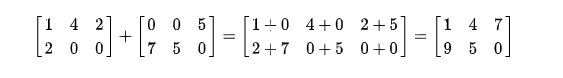矩阵的加法满足下列运算律(A，B，C都是同型矩阵)：
A+B = B+A
（A+B）+C = A+（B+C）
矩阵减法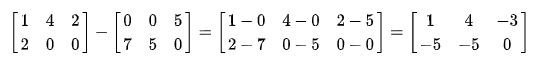矩阵数乘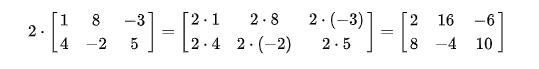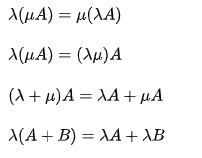矩阵转置
把矩阵A的行和列互相交换所产生的矩阵称为A的转置矩阵(A)T  ，这一过程称为矩阵的转置。
矩阵的转置满足以下运算律：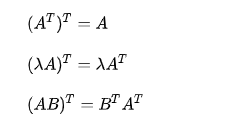矩阵乘法
两个矩阵的乘法仅当第一个矩阵A的列数和另一个矩阵B的行数相等时才能定义。如A是m×n矩阵和B是n×p矩阵，它们的乘积C是一个m×p矩阵 。乘积：C=AB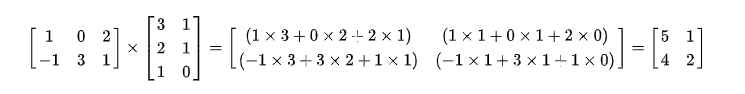矩阵的乘法满足以下运算律：
结合律：A(BC)=(AB)C
左分配律：A(B+C)=AB+AC
右分配律：(A+B)C=AC+BC
注意：矩阵乘法不满足交换律。
矩阵代码
public class MatrixTest {

public static void main(String[] args) {
// TODO Auto-generated method stub
//创建矩阵
int[][] a = new int;
int[][] b = new int;
int[][] c = new int;
//矩阵赋值
a=Matrix_value(a);
b=Matrix_value(b);
//输出矩阵
System.out.println("============== 矩阵A ==================");
Matrix_Show(a);
System.out.println("============== 矩阵B ==================");
Matrix_Show(b);
// 加法
System.out.println("============== 矩阵A + 矩阵B ============");
// 减法
System.out.println("============== 矩阵A - 矩阵B ============");
Matrix_Sub(a, b);
// 乘法
c=Matrix_value(c);
System.out.println("============== 矩阵C ==================");
Matrix_Show(c);
System.out.println("============== 矩阵A * 矩阵C ============");
Matrix_Mul(a, c);
//数乘
System.out.println("============== 2 * 矩阵A =================");
Matrix_Mul(2, a);
//转置
System.out.println("============== 矩阵A转置 =====================");
Matrix_Tran(a);
//旋转
System.out.println("============== 矩阵旋转  =====================");
for (int i = 1; i <= 3; i++) {
System.out.println("============== 旋转 " + i + " 次（每次旋转90度）  =====================");
a = Matrix_Rotate(a);
Matrix_Show(a);
}
//对角线之差
System.out.println("============== 矩阵对角线之差  =====================");
Matrix_Diag(a);
}

// 矩阵赋值
public static int[][] Matrix_value(int[][] a) {
for (int i = 0; i < a.length; i++) {
for (int j = 0; j < a.length; j++) {
a[i][j] = (int) (Math.random() * 100 + 1);
}
}
return a;
}

// 输出矩阵
public static void Matrix_Show(int[][] a) {
// System.out.println(Arrays.deepToString(a));
for (int i = 0; i < a.length; i++) {
for (int j = 0; j < a.length; j++) {
System.out.print(a[i][j] + " ");
}
System.out.println();
}

}

// 加法
public static void Matrix_Add(int[][] a, int[][] b) {
int[][] result = new int[a.length][a.length];
for (int i = 0; i < result.length; i++) {
for (int j = 0; j < result.length; j++) {
result[i][j] = a[i][j] + b[i][j];
}
}
Matrix_Show(result);

}

// 减法
public static void Matrix_Sub(int[][] a, int[][] b) {
int[][] result = new int[a.length][a.length];
for (int i = 0; i < result.length; i++) {
for (int j = 0; j < result.length; j++) {
result[i][j] = a[i][j] - b[i][j];
}
}
Matrix_Show(result);

}

// 乘法
public static void Matrix_Mul(int[][] a, int[][] b) {
int[][] result = new int[a.length][b.length];
for (int i = 0; i < result.length; i++) {
for (int j = 0; j < result.length; j++) {
for (int k = 0; k < b.length; k++) {
result[i][j] += a[i][k] * b[k][j];
}
}
}
Matrix_Show(result);
}

// 数乘
public static void Matrix_Mul(int n, int[][] a) {
int[][] result = new int[a.length][a.length];
for (int i = 0; i < result.length; i++) {
for (int j = 0; j < result.length; j++) {
result[i][j] = a[i][j] * n;
}
}
Matrix_Show(result);
}

// 矩阵转置
public static void Matrix_Tran(int[][] a) {
int[][] result = new int[a.length][a.length];
for (int i = 0; i < result.length; i++) {
for (int j = 0; j < result.length; j++) {
result[i][j] = a[j][i];
}
}
Matrix_Show(result);
}

// 矩阵旋转
public static int[][] Matrix_Rotate(int[][] a) {

// 获得原矩阵的宽高
// 外层一维数组的长度
int w = a.length;
// 内层一维数组的长度
int h = a.length;

// 创建一个新二维数组
int[][] c = new int[h][w];

// 原数组的读取位置
int x = w - 1;
int y = 0;

// 将原的二维数组反转90度，赋值到新的二维数组中
for (int i = 0; i < c.length; i++) {
for (int j = 0; j < c[i].length; j++) {
c[i][j] = a[x][y];
x--;
}

// 换行赋值
x = w - 1;
y++;
}

// 旋转90度之后的结果
return c;
}

// 矩阵对角线之差
public static void Matrix_Diag(int[][] a) {
// 主对角线
int x = 0;
// 次对角线
int y = 0;
// 外层一维数组的长度
int i = 0;
// 内层一维数组的长度
int j = 0;
// 求主对角线值
while ((i < a.length) && (j < a.length)) {
x += a[i][j];
i++;
j++;
}
i = 0;
j = a.length - 1;
// 求次对角线值
while ((i < a.length) && (j >= 0)) {
y += a[i][j];
i++;
j--;
}
System.out.println("矩阵对角线之差为 " + (x - y));
}
}

文章图片来源
百度-矩阵


展开全文线性代数
• 我正在尝试用Java计算逆矩阵.我正在遵循伴随方法(首先计算伴随矩阵,然后转置这个矩阵,最后,将它乘以行列式值倒数).当矩阵不太大时,它可以工作.我已经检查过,对于尺寸为12×12的矩阵,可以快速得到结果.但是,当矩阵...
我正在尝试用Java计算逆矩阵.我正在遵循伴随方法(首先计算伴随矩阵,然后转置这个矩阵,最后,将它乘以行列式值的倒数).当矩阵不太大时,它可以工作.我已经检查过,对于尺寸为12×12的矩阵,可以快速得到结果.但是,当矩阵大于12×12时,完成计算所需的时间呈指数增长.我需要反转的矩阵是19×19,需要花费太多时间.更多时间消耗的方法是用于计算行列式的方法.我正在使用的代码是：public static double determinant(double[][] input) {int rows = nRows(input);        //number of rows in the matrixint columns = nColumns(input); //number of columns in the matrixdouble determinant = 0;if ((rows== 1) && (columns == 1)) return input;int sign = 1;for (int column = 0; column < columns; column++) {double[][] submatrix = getSubmatrix(input, rows, columns,column);determinant = determinant + sign*input[column]*determinant(submatrix);sign*=-1;}return determinant;}有人知道如何更有效地计算大矩阵的行列式吗？如果没有,有没有人知道如何使用其他算法计算大矩阵的逆？谢谢解决方法:成倍？不,我相信矩阵求逆是O(N ^ 3).我建议使用LU decomposition来求解矩阵方程.使用它时,您无需为行列式求解.更好的是,查看一个包来帮助你.想到了JAMA.12×12或19×19不是大型matricies.解决具有数十或数十万自由度的问题是很常见的.这是一个如何使用JAMA的工作示例.编译和运行时,必须在CLASSPATH中使用JAMA JAR：package linearalgebra;import Jama.LUDecomposition;import Jama.Matrix;public class JamaDemo{public static void main(String[] args){double [][] values = {{1, 1, 2}, {2, 4, -3}, {3, 6, -5}};  // each array is a row in the matrixdouble [] rhs = { 9, 1, 0 }; // rhs vectordouble [] answer = { 1, 2, 3 }; // this is the answer that you should get.Matrix a = new Matrix(values);a.print(10, 2);LUDecomposition luDecomposition = new LUDecomposition(a);luDecomposition.getL().print(10, 2); // lower matrixluDecomposition.getU().print(10, 2); // upper matrixMatrix b = new Matrix(rhs, rhs.length);Matrix x = luDecomposition.solve(b); // solve Ax = b for the unknown vector xx.print(10, 2); // print the solutionMatrix residual = a.times(x).minus(b); // calculate the residual errordouble rnorm = residual.normInf(); // get the max error (yes, it's very small)System.out.println("residual: " + rnorm);}}根据quant_dev的建议,使用Apache Commons Math解决了同样的问题：package linearalgebra;import org.apache.commons.math.linear.Array2DRowRealMatrix;import org.apache.commons.math.linear.ArrayRealVector;import org.apache.commons.math.linear.DecompositionSolver;import org.apache.commons.math.linear.LUDecompositionImpl;import org.apache.commons.math.linear.RealMatrix;import org.apache.commons.math.linear.RealVector;public class LinearAlgebraDemo{public static void main(String[] args){double [][] values = {{1, 1, 2}, {2, 4, -3}, {3, 6, -5}};double [] rhs = { 9, 1, 0 };RealMatrix a = new Array2DRowRealMatrix(values);System.out.println("a matrix: " + a);DecompositionSolver solver = new LUDecompositionImpl(a).getSolver();RealVector b = new ArrayRealVector(rhs);RealVector x = solver.solve(b);System.out.println("solution x: " + x);;RealVector residual = a.operate(x).subtract(b);double rnorm = residual.getLInfNorm();System.out.println("residual: " + rnorm);}}根据您的情况调整这些.标签：matrix-inverse,java,matrix,determinants来源： https://codeday.me/bug/20190926/1818581.html
展开全文• 我需要在我应用程序中使用矩阵类进行操作，如特征值分解，LUDecomposition以及正常的矩阵运算，如乘法，加法，换位等 . 我决定使用jama，因为我对它非常熟悉，因为我目前使用小数据集 .但可能以后，随着数据量...
我需要在我的应用程序中使用矩阵类进行操作，如特征值分解，LUDecomposition以及正常的矩阵运算，如乘法，加法，换位等 . 我决定使用jama，因为我对它非常熟悉，因为我目前使用小数据集 .但可能以后，随着数据量的增长，我希望转向更好的矩阵库，如ejml或一些未来的发明 . 我应该如何设计我的矩阵类，使其不受特定实现的约束？我想要一个类似于下面的界面 .class MyMatrix{public MyMatrix addWithAnother(MyMatrix b){...}public MyMatrix multiplyWithAnother(MyMatrix b){...}public double[][] getData(){..}public SomeEigenDecomposition getEigenValueDecomposition(){...}我应该将实现矩阵类(jama Matrix或类似)作为字段并委托调用吗？我认为，这仍然会让我与jama联系在一起 .如何让上面的内容完全免于实现？任何设计模式，java大师请指教 .谢谢标记
展开全文• 已知一个NxN的矩阵A,求矩阵中所有边长为m正方形子矩阵内元素中位数。(m例子: // m = 3 n = 5// 01234݂// 0 11357݂// 1 29429݂// 2 38668݂// 3 47851݂// 4 56342݂// 01234݂...
已知一个NxN的矩阵A,求矩阵中所有边长为m的正方形的子矩阵内元素的中位数。(m例子: // m = 3 n = 5// 01234݂         //  0 11357݂         //  1 29429݂         //  2 38668݂         //  3 47851݂         //  4 56342݂         //  01234݂         //  @@@##݂         //  @@@##݂         //  @@@##݂         //  #####݂         //  #####݂         //  标准答案应该是݂         //  356݂         //  666݂         //  665情况1:m的范围在[1, 10]情况2:m范围不限数值范围在[1, 10]情况3:m范围不限数值范围不限vector> getMidVal(const vector> &mat, int m);
展开全文• 展开全部packagetest;importjava.util..../***java-用邻接矩阵求图最短路径、最长途径。弗洛伊德算法32313133353236313431303231363533e58685e5aeb931333361313961*/publicclassFloydInGraph{privatestati...
• 本文实例讲述了Java实现打印螺旋矩阵算法。分享给大家供大家参考，具体如下：一个N阶矩阵顺时针呈螺旋状，形如下面的矩阵：思路：使用一个二维数组存放数据，数组初始化为0，数组共有n平方个元素，因为是从1开始，...
• public class TestArr {public static void main(String[] args) {int m = 3; // m行int n = 4;... // m行n列矩阵 = m个长度为n数组 = 3个长度为4数组System.out.println("arr.length = " + arr.len...
• 今天对Java的类有了简单认识，按照书上习题编写了一个矩阵类。 题目：尝试编写一个矩阵类，将长和宽作为矩阵属性，在构造方法中将、宽初始化，定义一个成员方法求次矩形面积。 自己写代码如下： public ...
• 泛型概述C#中泛型C#泛型和java泛型比较C#泛型和C++模板比较C#泛型中约束泛型概述Bruce Eckel：您能对泛型做一个快速介绍么？Anders Hejlsberg： 泛型其实就是能够向你类型中加入类型参数一种能力，也...
• 记得以前一次程序题，花好时间才弄出来：给定一个正整数n，构造一个n×n维的矩阵，从1，2，........到n×n使其位置轨迹构成一个螺旋状,for example when n=5,the matrix showed as below:1 , 2 ,3 ,4 ,5 ,16...
• 1 基本数据类型byte：Java中最小数据类型，在内存中占1个字节(8 bit)，取值范围-128~127，默认值0short：短整型，2个字节(16 bit)，取值范围-32768~32717，默认值0int：整型，用于存储整数，在内存中占4个字节，...
• 本例输入为两个任意尺寸的矩阵m * n, n * m，输出为两个矩阵的乘积。计算任意尺寸矩阵相乘时，使用了Strassen算法。程序为自编，经过测试，请放心使用。基本算法是：1.对于方阵(正方形矩阵)，找到最大的l, 使得l = 2...
• ## Java中的矩阵使用

千次阅读 2016-09-20 19:39:02
public class TestArr { public static void main(String[] args) { int m = 3; // m行 int n = 4; // n列 int[][] arr = new int[m][n]; // m行n列矩阵 = m个长度为n数组 = 3个长度为4数组 Sys
• Java中ArrayList类详细介绍ArrayList是一个可变长度数组，它实现了List接口，因此它也可以包含重复元素和Null元素，也可以任意访问和修改元素，随着向 ArrayList 中不断添加元素，其容量也自动增长。不过ArrayList...
• 本例输入为两个任意尺寸的矩阵m * n, n * m，输出为两个矩阵的乘积。计算任意尺寸矩阵相乘时，使用了Strassen算法。程序为自编，经过测试，请放心使用。基本算法是：1.对于方阵(正方形矩阵)，找到最大的l, 使得l = 2...
• 本文实例讲述了Java实现打印螺旋矩阵算法。分享给大家供大家参考，具体如下：一个N阶矩阵顺时针呈螺旋状，形如下面的矩阵：思路：使用一个二维数组存放数据，数组初始化为0，数组共有n平方个元素，因为是从1开始，...
• 我目前方法似乎非常低效，导致非常长的处理时间(例如，使用具有52,000个长度为500向量向量集需要~36小时来构建52,000x52,000矩阵) .我目前解决方案使用是R版3.0.1(2013-05-16)，在英...
• 题目描述还记得童话《卖火柴小女孩》吗？现在，你知道小女孩有多少根火柴，请找出一种能使用所有火柴拼成一个正方形方法。不能折断火柴，可以把火柴连接起来，并且每根火柴都要用到。输入为小女孩拥有火柴数目...
• 数组是Java一种容器对象，它拥有多个单一类型值。当数组被创建时候数组长度就已经确定了。在创建之后，其长度是固定。下面是一个长度为10数组：public class ArrayDemo {private int arraySize=10;...
• 写一个函数，给定矩阵的长度级数n,返回一个回旋排列的数字矩阵:例如:n=2返回:1 23 4n=3返回：1 2 34 5 67 8 9import numpydef Matrix():N = 4M = Narray = numpy.zeros((N, M), dtype=numpy.int16)# 起始点x, y = .....
• 分享给大家供大家参考，具体如下：题目：按照指定的长宽和输出方向，从外向内打印一个从 1 开始的数字矩阵，矩阵的开始位置在左上角。如下图代码及注释如下：public class NumberMatrix {public static void main...
• 算法思想：分治法 实际问题：Strassen 矩阵乘法 编写语言：Java问题描述我们知道，两个大小为 2 * 2 的矩阵相乘，一般需要进行 8 次乘法。而Strassen矩阵乘法可以减少一次乘法，只需要 7 次，看似很少，但当数据量很...
• ## 螺旋矩阵的Java实现

千次阅读 2007-11-04 21:32:00
记得以前一次程序题，花好时间才弄出来：给定一个正整数n，构造一个n×n维的矩阵，从1，2，........到n×n使其位置轨迹构成一个螺旋状,for example when n=5,the matrix showed as below:1 , 2 , 3 , 4 ,...string matrix command
• 329. 矩阵最长递增路径 给定一个整数矩阵，找出最长递增路径长度。 对于每个单元格，你可以往上，下，左，右四个方向移动。 你不能在对角线方向上移动或移动到边界外（即不允许环绕）。 示例 1: 输入: nums = ...
• 矩阵最长递增路径 https://leetcode-cn.com/problems/longest-increasing-path-in-a-matrix/ 给定一个整数矩阵，找出最长递增路径长度。 对于每个单元格，你可以往上，下，左，右四个方向移动。 你不能在对...leetcode 算法 dfs 动态规划
• 有没有办法平均每个矩阵的第一行，并输出到一个新的矩阵，其中第一个单元格是所有矩阵第一个单元格的平均值？然后第二个和第三个一直到最后？到目前为止，这是我的脚本 . 你可以看到我创建了一个矩阵 . 现在我想......

# java矩阵的长java 订阅